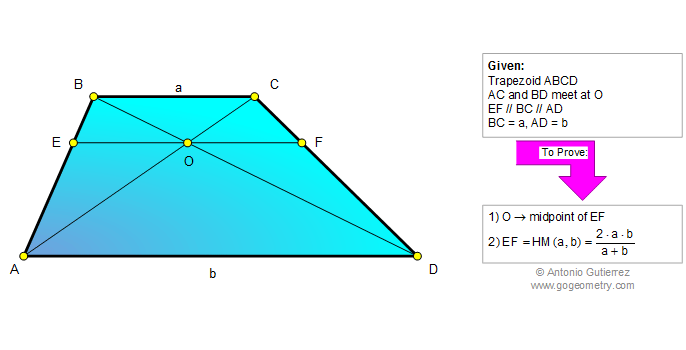# Geometry Problem 747: Unraveling the Properties of Trapezoids with Intersecting Diagonals, Exploring Parallel Bases, Midpoints, Similarity, and Harmonic Mean

< PREVIOUS PROBLEM  |  NEXT PROBLEM >

The figure shows a trapezoid ABCD with the diagonals AC and BD meeting at O. EF, passing through O, is parallel to the bases AD and BC. Prove that (1) O is the midpoint of EF, (2) EF is the harmonic mean of AD and BC.## Thematic Poem: Exploring the Secrets of Trapezoids: Unraveling Properties with Intersecting Diagonals, Midpoints, Similarity, and Harmonic Mean

A trapezoid with intersecting diagonals,
A challenge posed to the mathematical royals,
To unravel the properties of this shape,
And reveal the secrets it doth drape.

Through the midpoint O, the diagonals meet,
EF parallel to bases, adds to the feat,
To prove that O divides EF in two,
Is a task that requires an insightful view.

With similar triangles, the path we tread,
And the parallel lines that we are led,
To the midpoint we prove that we have arrived,
And a property of the trapezoid we have derived.

But the journey's not done, the challenge remains,
To find the harmonic mean, and reap the gains,
Of a deeper understanding of this shape,
And the knowledge that it doth create.

Through similarity, we find a way,
To express the lengths, and make them play,
With the harmonic mean, a value so grand,
A new property of the trapezoid, we understand.

So we unravel the properties of this shape,
Exploring parallel bases, midpoints, and the tape,
That binds together the secrets it holds,
And reveals to us, a world so bold.